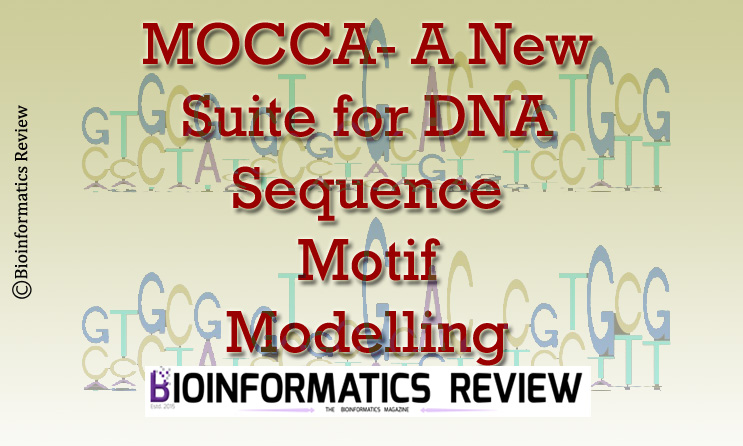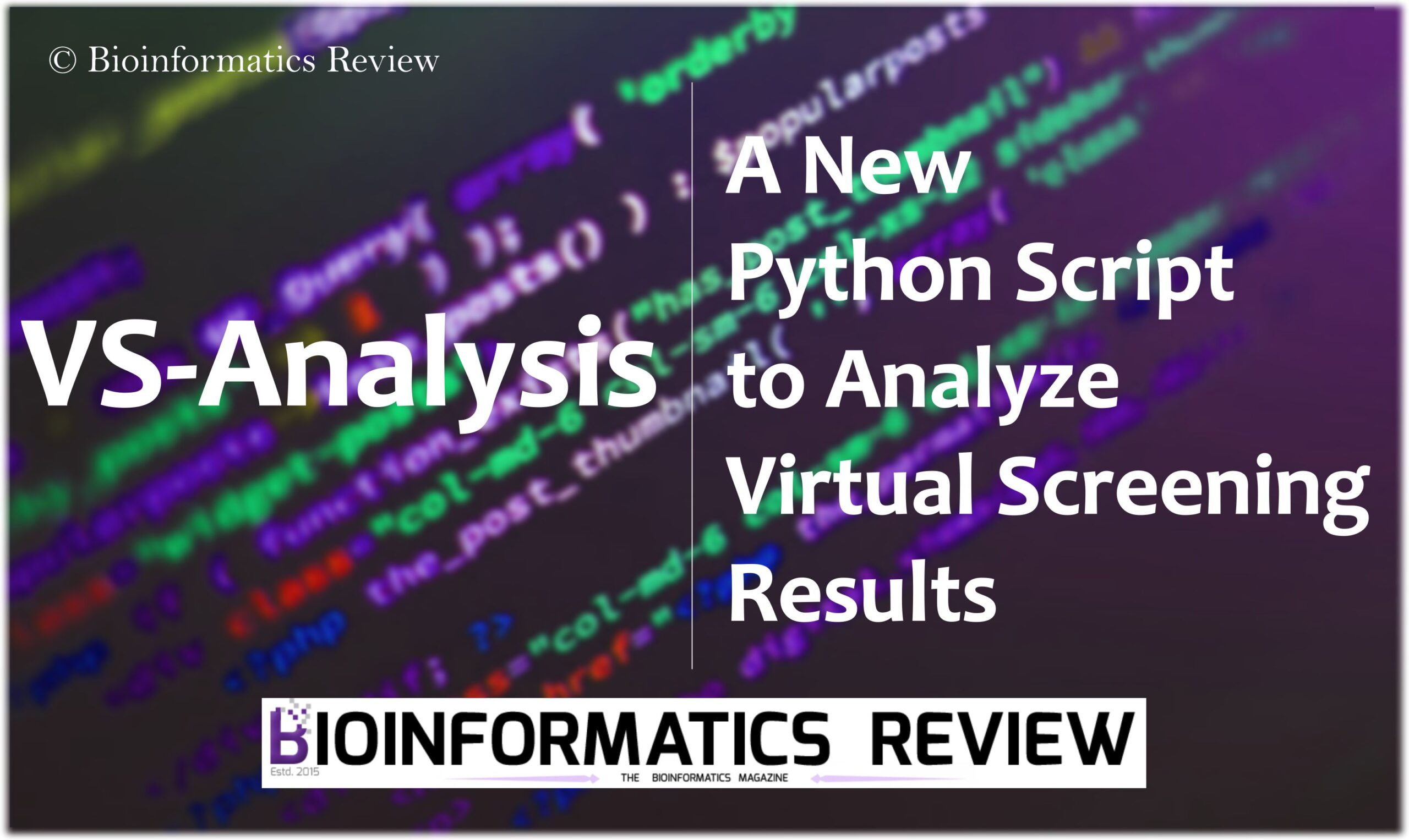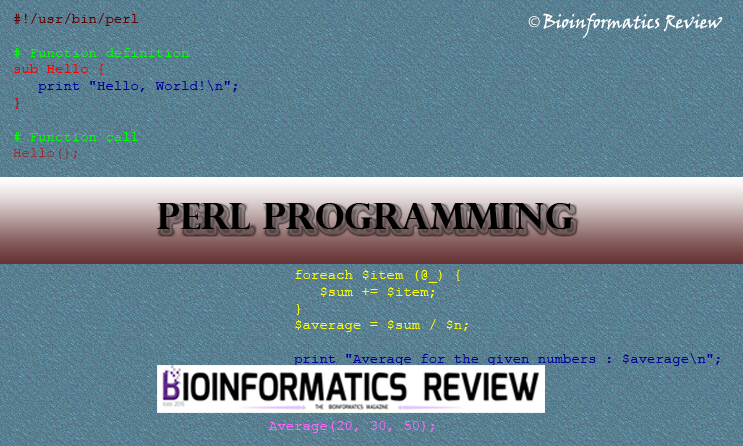# Role of Information Theory, Chaos Theory, and Linear Algebra and Statistics in the development of alignment-free sequence analysisPublished

onSequence alignment is customary to not only find similar regions among a pair of sequences but also to study the structural, functional and evolutionary relationship between organisms. Many tools have been discovered to achieve the goal of alignment of a pair of sequences, separately for nucleotide sequence and amino acid sequence, BLOSSUM & PAM  are a few to name. There are many methods of alignment such as pairwise alignment. Multiple sequence alignment, on the other hand, is used for aligning 3 or more sequences. It is considered as the first step in phylogenetic studies. Progressive alignment is the base for developing various multiple alignment tools. For the validation of alignment, benchmark datasets are used. One tool that has been extensively used for this purpose is BaliBASE . It is a database of refined multiple sequence alignments consisting of high quality documented alignments to identify the strong and weak points of the numerous alignment programs IRMBase , SABMark , OXBENCH  are a few to name.

The limitations that led to the development of algorithm for alignment-free sequence analysis are 1) incompleteness in approach to sequence divergence and also reflects conservation of contiguity between homologous segments, 2) unfeasibility in searching large databases as a result of escalation in computational load being considered as a power function, 3) heuristic solutions make it harder to assess the statistical relevance of the resulting scores which compromises the establishment of confidence intervals for homology.

Various physics methods, mathematical modeling techniques such as Information Theory, Chaos Theory, Linear Algebra, and Statistics have been used to achieve the aim [5,6,7,8,9,10,11]. As the sequence data is increasing exponentially it is unfeasible to use alignment-based methods for distinctly related sequences. Implementation of the idea of alignment-free methods previously done worldwide in various fields such as phylogenomics, NGS, epigenomics, SNP discovery etc., have been discussed briefly in this paper. Diogo Pratas et al (2015)  has described an alignment-free computational method, based on blind unsupervised approach, to detect large-scale and small-scale genomic rearrangements between pairs of DNA sequence. Cheon Xin Chan (2013) discussed k-mer method . Shea N Gardner and Barry G. Hall (2013)  have explained about a software called kSNP v2 for alignment-free SNP and phylogenetics. MAUVE, Cinteny, Apollo, Mizbee are a few tools for visualization purposes to name . Some other well-known websites or software developed for alignment-free methods are kmacs , Spaced words , and rush .

Linear Algebra & Statistics

For the sequences to be considered as objects or vectors, mathematical techniques have been used . By scaling up the vectors N number of sequence combinations could be derived. Euclidean distance has been used to achieve complete independence from the contiguity of conserved segments and find the difference between sequences.  The same method has also been used to calculate the correlation among the sequences. To calculate the covariance between sequences, another metric method called Mahalanobis Distance method is used which indicates the extent to which prefix and suffix of a word are equal between a point and a distribution. Statistical significance of the sequence comparison is assessed by Chi-square test . There are many method frames based on k-mer/ word frequency, Feature frequency profile (FFP), Composition Vector, Return time distribution (RTD) are to name a few [18,19,20,21].

FFP method works by calculating the count of each possible k-mer in sequence [18, 19]. A k-mer is a unit of information, in this case, it is the nucleotides or amino acids present in the sequence. Each k-mer count in each sequence is then normalized by dividing it by total of all k-mer’s count in that sequence, therefore converting each sequence into its feature frequency profile. Jensen-Shannon divergence (JSD) is a method of measuring the similarity between two probability distributions, otherwise called as information radius (iRad) or total divergence to the average . The equation defining JSD is:

`JSD(P||Q) = 1/2D(P||M) + 1/2D(Q||M)`

where,

M = ½(P+Q).

This divergence method is used to calculate the pairwise distance between two sequences. The resulting distance matrix can then be used to construct a phylogenetic tree using clustering algorithms.

In the composition of the vector method, the frequency of the appearance of each possible k-mer in the sequence is calculated. Markov model is used to reduce the influence of random neutral mutations to highlight the role of selective evolution. Composition vector (CV) of a given sequence is then formed by normalizing the frequencies and putting them in a fixed order. The pairwise distance of CVs of given sequences is then computed using cosine distance. In general, cosine distance is a measure of similarity between two non-zero vectors of an inner product space that measures the cosine angle between them.

`distance = cos-1 (similarity)/ π`

Where,

The above equation defines the cosine distance when the vector elements are positive.  The resultant matrix is used to construct a tree using clustering algorithms [23,24].  Instead of calculating the count of k-mers as done by previously discussed methods, the RTD method computes the time required for the reappearance of k-mers. The values are summarized using two statistical parameters mean and standard deviation. The pairwise distance is calculated using Euclidean distance and the last step is the construction of tree using clustering algorithms.

Information Theory

The information is a vital part of all forms of communication which is measured in bits . The equation framed for quantifying the capability of transmission of data over a channel has been used to calculate probabilities of different outcomes in sequence comparison. The equation is:

`H(WXL) = -Σi=1K PXL,i log2(pXL,i)`

In simple words, the longer the length of the sequence is, the more complex the object is considered. Kolmogorov’s complexity is hence used to reconstruct the given sequence .

Global and local characterization of DNA, RNA, proteins, estimation of genome entropy to motif and region classification are some of the existing applications of Information Theory in building alignment-free methods.

Some of the principles used to develop based on information theory in developing software are here under.

a) Base-base correlation: converts the genome sequence into 16-dimensional numeric vectors using the following equation:

`Tij(K) = ΣK Pij(l).log2`
where,

Pi  and Pj denote probabilities of the bases i and j in the genome.

Pij(l) indicates the probabilities of bases i and j at the distance l in the genome.

parameter K indicates the maximum distance between the bases i and j.

The variation in the values of 16 parameters reflects variation in the genome content and length [25,26,27].

b) Information correlation:  partial information correlation: This method employs the base correlation property of the DNA sequence. IC and PIC were calculated using the below formulas:

`ICi = -2ΣiPilog2Pi + ΣijPij(l)log2Pij(l)`
`PICij(l) = [Pij(l) - PiPj (l)]2`
`V ICl/PICij(l) where l ∈ {l0,l0+1,...l0+n}`

The final vector obtained defines the range of distance between bases. Euclidean distance is used to calculate the pairwise distance between sequences and the distance matrix is used to construct a tree using clustering algorithms .

c) Context modeling compress: This method was described by Pinho et al., (2013) . It is a method which is used in DNA sequence analysis. In this method, the next symbol predictions, of one or more statistical models are combined to yield a prediction that is based on events that are recorded in the past. The algorithmic information derived from each symbol prediction can be used to compute algorithmic information profiles with a time proportional to the length of the sequence.

Universal Sequence Map (Chaos Theory)

The proposition of iterative maps or iterative functions for the representation of DNA is considered similar to that of one of the principles of Chaos Theory, Fractal [30, 31]. The coordinate position of each unit of a sequence of nucleotide or amino acid that defines the trajectories in continuous space encodes for both its identity and its context.

Mathematically, the chaos game is described by an iterated function system. An IFS is a set of pairs of linear equations, each pair of the form:

`x = ax+by+e, y = cx+dy+f`

Each pair of equations gives the formula for computing the new value of x and y coordinates. This was the period iterative maps introduced by HJ Jefferey .

This representation defines a unit square where each corner corresponds to one of the four possible nucleotides [6,7]. Due to the lack of scalability with regard to the number of possible unique units and inability to represent succession schemes, Markov models have been used for the identification of discrete spaces to represent sequences as cross-tabulated conditional probabilities –Markov Transition tables .

To measure the homology and to align sequences Bayesian theory has been used. Therefore the use of iterative maps has been found to be both essential and effective not only for representation of sequences but also for identifying scale independent stochastic models of the succession schemes . A number of web pages such as GitHub  are available to demonstrate how to encode and compare arbitrary symbolic sequences. MapReduce is also being utilized for the same purpose . MapReduce coding pattern is most widely used as it finds natural distribution via map functions to process vectorized components and reduction of aggregate intermediate results.

#### Conclusion

Multiple sequence alignment being heuristic in nature reflects methodology incompleteness in approach to sequence divergence and also reflects conservation of contiguity between homologous segments. The percentage of unfeasibility in searching large databases as a result of the escalation in computational load increases when using heuristic solutions. Assessment of statistical scores which compromises the establishment of confidence intervals for homology becomes harder.

Alignment-based methods require substitution or evolutionary models and are expensive as they rely on dynamic programming to find the alignment that has an optimal score. On the other hand, alignment-free methods do not assume continuity of homologous regions. It is computationally inexpensive and memory intensive, less dependent on substitution or evolutionary models. Unlike alignment-based methods these are less sensitive to stochastic sequence variation, recombination, horizontal gene transfer etc., which is time efficient. Indexing word counts or positions in fractal space are the alternatives to dynamic programming used in alignment-based methods. An algorithm satisfying the need of an alignment-free program for sequence analysis would be a good solution to overcome all the limitations of the already developed programs in use.

#### Discussion and future developments

The awareness developed by the existence and importance of alignment-free methods would help the scientific community to develop more efficient tools to overcome the limitations faced while handling alignment-based methods.

The current trend is the use of MapReduce to analyze the sequence data. Proper understanding and implementation of alignment-free methods could be used more efficiently in the area of metagenomics, for phylogeny reconstruction, protein classification and finally in decoding the sequence information eventually helping in studying disease patterns. The need of accurate alignments without compromising their specificity and sensitivity is increased thereby, increasing the demand for new algorithms.

#### References

1. Robert C Edgar1 and Serafim Batzoglou2 Multiple Sequence Alignment
2. D. Thompson, Frederic Plewniak and Oliver Poch (1999). BAliBASE: a benchmark alignment database for the evaluation of multiple alignment programs.
3. Amarendran R Subramanian, Jan Weyer-Menkhoff, Michael Kaufmann and Burkhard MorgensternEmail author BMC Bioinformatics20056:66 DOI: 10.1186/1471-2105-6-66
4. Ivo Van Walle Ignace Lasters  Lode Wyns Bioinformatics (2005) 21 (7): 1267-1268. DOI: https://doi.org/10.1093/bioinformatics/bth493
5. Raghava, G. P. S., Searle, S. M. J., Audley, P. C., Barber, J. D., & Barton, G. J. (2003). OXBench: a benchmark for evaluation of protein multiple sequence alignment accuracy. BMC Bioinformatics, 4, 47.
6. Shannon, C.E. (1948) A mathematical theory of The Bell System Technical J., 27, 379–423, 623–656.
7. Jeffrey HJ: Chaos game representation of gene structure. Nucleic Acid Res. 1990, 18: 2163–2170.
8. Goldman N.: Nucleotide, dinucleotide and trinucleotide frequencies explain patterns observed in chaos game representations of DNA sequences. Nucleic Acids Res. 1993, 21: 2487–2491.
9. Almeida, J.S. & Vinga, S. BMC Bioinformatics (2002) 3: 6. doi:10.1186/1471-2105-3-6 Universal Sequence Maps of arbitrary discrete sequences
10. Gr¨unwald, P. and P. Vit´anyi (2005). Shannon information and Kolmogorov complexity.
11. Strang,G. (1988) Linear Algebra and Its Applications. Thomson, London.
12. Schott,J.R. (1997) Matrix Analysis for Statistics. Wiley, New York
13. Pratas, D., Silva, R. M., Pinho, A. J., & Ferreira, P. J. S. G. (2015). An alignment-free method to find and visualise rearrangements between pairs of DNA sequences. Scientific Reports, 5, 10203.
14. Chan, C. X., & Ragan, M. A. (2013). Next-generation phylogenomics. Biology Direct, 8, 3.
15. Gardner, S. N., & Hall, B. G. (2013). When Whole-Genome Alignments Just Won’t Work: kSNP v2 Software for Alignment-Free SNP Discovery and Phylogenetics of Hundreds of Microbial Genomes. PLoS ONE, 8(12), e81760.
16. Leimeister, C.-A., & Morgenstern, B. (2014). kmacs: the k-mismatch average common substring approach to alignment-free sequence comparison. Bioinformatics, 30(14), 2000–2008
17. Chris-Andre Leimeister, Marcus Boden, Sebastian Horwege, Sebastian Lindner, Burkhard Morgenstern; Fast alignment-free sequence comparison using spaced-word frequencies.
18. Bernhard Haubold, Linda Krause, Thomas Horn, Peter Pfaffelhuber; An alignment-free test for recombination. Bioinformatics 2013; 29 (24): 3121-3127. doi: 10.1093/bioinformatics/btt550
19. Sims, G. E., Jun, S.-R., Wu, G. A., & Kim, S.-H. (2009). Whole-genome phylogeny of mammals: Evolutionary information in genic and nongenic regions. Proceedings of the National Academy of Sciences of the United States of America, 106(40), 17077–17082.
20. Sims, G. E., & Kim, S.-H. (2011). Whole-genome phylogeny of Escherichia coli/Shigella group by feature frequency profiles (FFPs). Proceedings of the National Academy of Sciences of the United States of America, 108(20), 8329–8334.
21. Graham L. Giller (2012). “The Statistical Properties of Random Bitstreams and the Sampling Distribution of Cosine Similarity”. Giller Investments Research Notes (20121024/1).
22. Alignment-free distance measure based on return time distribution for sequence analysis: Applications to clustering, molecular phylogeny and subtyping Pandurang Kolekara, , Mohan Kaleb, , Urmila Kulkarni-Kalea
23. http://www.abarim-publications.com/ChaosTheoryIntroduction.html#.WJbzMlN97IU
24. Apostolico, A; Denas, O; Dress, A (September 2010). “Efficient tools for comparative substring analysis.”. Journal of Biotechnology. 149 (3): 120–126. doi:10.1016/j.jbiotec.2010.05.006
25. Apostolico, A; Denas, O (March 2008). “Fast algorithms for computing sequence distances by exhaustive substring composition.”. Algorithms for Molecular Biology.
26. . Cheng, J., Zeng, X., Ren, G., & Liu, Z. (2013). CGAP: a new comprehensive platform for the comparative analysis of chloroplast genomes. BMC Bioinformatics, 14, 95.
27. Coronavirus phylogeny based on Base-Base Correlation Zhi-Hua LiuRelated information1 State Key Laboratory of Bioelectronics, Southeast University, Nanjing 210096, PR China; Harvard Medical School, Dana-Farber Cancer Institute, Department of Biostatistics and Computational Biology, 44 Binney St., Boston, Massachusetts 02115, USA; Harvard School of Public Health, 677 Huntington Avenue, Boston, Massachusetts 02115, USA., Xiao SunRelated information2 State Key Laboratory of Bioelectronics, Southeast University, Nanjing 210096, PR China
28. A novel feature-based method for whole genome phylogenetic analysis without alignment: Application to HEV genotyping and subtyping Zhihua Liua, b, c, , , , Jihong Mengd, Xiao Suna.
29. Genome-based phylogeny of dsDNA viruses by a novel alignment-free method. Gao Y1, Luo L
30. Pinho, A. J., Garcia, S. P., Pratas, D., & Ferreira, P. J. S. G. (2013). DNA Sequences at a Glance. PLoS ONE, 8(11), e79922.
31. http://fractalfoundation.org/resources/what-is-chaos-theory/
32. http://usm.github.com/
33. Almeida, J. S., Grüneberg, A., Maass, W., & Vinga, S. (2012). Fractal MapReduce decomposition of sequence alignment. Algorithms for Molecular Biology : AMB, 7, 1Click to comment

# MOCCA- A New Suite to Model cis- regulatory Elements for Motif Occurrence CombinatoricsPublished

oncis-regulatory elements are DNA sequence segments that regulate gene expression. cis-regulatory elements consist of some regions such as promoters, enhancers, and so on. These regions consist of specific sequence motifs. (more…)

# vs_Analysis.py: A Python Script to Analyze Virtual Screening Results of Autodock VinaPublished

onThe output files obtained as a result of virtual screening (VS) using Autodock Vina may be large in number. It is difficult or quite impossible to analyze them manually. Therefore, we are providing a Python script to fetch top results (i.e., compounds showing low binding affinities). (more…)

# How to search motif pattern in FASTA sequences using Perl hash?Published

onHere is a simple Perl script to search for motif patterns in a large FASTA file with multiple sequences.